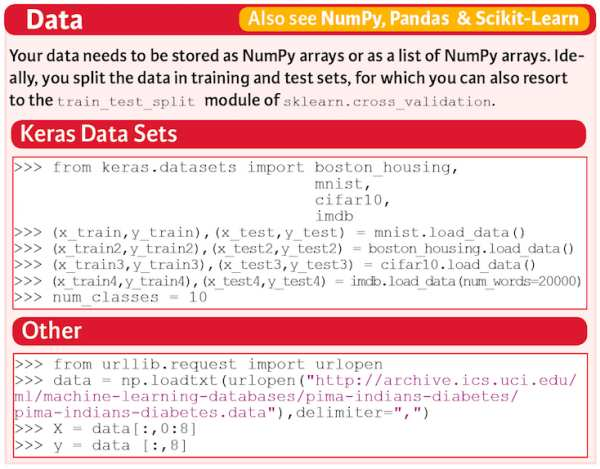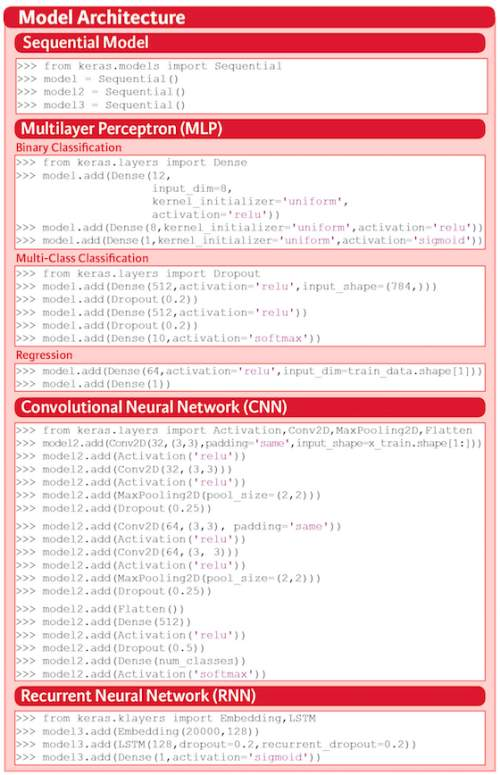# Keras Cheat Sheet: Deep Learning in Python

Keras is a Python deep learning library for Theano and TensorFlow. The package is easy to use and powerful, as it provides users with a high-level neural networks API to develop and evaluate deep learning models.

By Karlijn Willems, DataCamp.

### Deep Learning With Python

Deep learning is a very exciting subfield of machine learning that is a set of algorithms, inspired by the structure and function of the brain. These algorithms are usually called Artificial Neural Networks (ANN). Deep learning is one of the hottest fields in data science with many case studies with marvelous results in robotics, image recognition and Artificial Intelligence (AI).

This undoubtedly sounds very exciting (and it is!), but it is definitely one of the more complex topics in data science to get into. If you have prior machine learning experience, though, you should be getting started with deep learning pretty easily, as you will have already proven that you have understood, practiced and assimilated the necessary mathematics, statistics and machine learning basics. Maybe you have already worked on machine learning projects or you have even participated in a Kaggle or DrivenData competition!

However, even with this prior experience, you’ll still find that this complex topic is interestingly challenging! This doesn’t need to mean that you shouldn’t dive in any code straight away - You can also get a high-level idea how deep learning techniques work by using, for example, the Keras package. This package is ideal for beginners, as it offers you a high-level neural networks API with which you can develop and evaluate deep learning models easily and quickly.

Nevertheless, doubts may always arise and when they do, take a look at DataCamp’s, Keras tutorial or download the cheat sheet for free!

In what follows, we’ll dive deeper into the structure and the contents of the cheat sheet.

### Keras Cheat SheetStarting with Keras is not too hard if you take into account that there are some steps that you need to go through: gathering your data, preprocessing it, constructing your model, compiling and fitting your model, evaluating the model’s performance, making predictions and fine-tuning the model.

This might seem quite abstract. Let’s take a quick look at an example.

A very basic example in which the Keras library is used is to make a simple neural network with just one input and one output layer. To be able to build up your model, you need to import two modules from the Keras package: Sequential and Dense.

Next, you need some data. This example makes use of the random module of NumPy, the fundamental package for scientific computing in Python, to quickly generate some data and labels for you. That’s why you also import the numpy package with the conventional alias np. With the functions from the random module, you’ll first construct an array with size (1000,100). Next, you’ll also construct a labels array that consists of zeroes and ones and is of size (1000,1).

With the data at hand, you can start constructing your neural network architecture. A quick way to get started is to use the Keras Sequential model: it’s a linear stack of layers. You can easily create the model by passing a list of layer instances to the constructor, which you set up by running model = Sequential(). After that, you first add an input layer to the model with the add() function. You pick a dense or fully connected layer, where you indicate that you’re dealing with an input layer by using the argument input_dim. You also use one of the most common activation functions here -relu- and you pick 32 units for the input layer of your model. Next, you also add another dense layer as an output layer. It’s of size 1 with a sigmoid activation function to calculate the probabilities.

With the model built up, you can compile it with the help of the compile() function. you configure the model with the rmsprop optimizer and the binary_crossentropy loss function. Additionally, you can also monitor the accuracy during the training by passing ['accuracy'] to the metrics argument.

Next, you fit the model to the data with fit(): you pass in the data, the labels, set the number of epochs and the batch size. Lastly, you can finally start making predictions with the help of the predict() function. Just pass in the data!

Simple enough, right? Let’s take a look at all these steps in more detail.

### DataAs you might have gathered from the short example that was just covered in the first section, your data needs to be stored as a NumPy array or as a list of NumPy arrays in order to get started. Also, ideally, you split the data into training and test sets, which is something that was neglected in the example above. In such cases, you can resort to the train_test_split() function which you can find in the cross_validation module of Scikit-Learn, the library for machine learning in Python.

If you want to work with the data sets that come with the Keras library, you can easily do so by importing them from the datasets module. You can use the load_data() functions to get the data split in training and test sets, into your workspace. Alternatively, you can also use the urllib library and its request module to open and read URLs.

### Preprocessing

Now that you have the data, you can easily proceed to preprocessing it. Of course, depending on your data, you’ll need to resort to different functions to make sure that the data looks exactly the way it needs to look to pass it to the neural network model.For example, you can use sequence padding with pad_sequences() to ensure that all sequences in a list have the same length, or you can use one-hot encoding with to_categorical() to generate one boolean column for each categorical feature. These functions come with the Keras library.

However, as mentioned before, you will most probably also need to resort to other libraries for preprocessing - Think of the train and test set splits, or the standardization/normalization functions that come with the Scikit-Learn library. If you’d like to know more, take a look at the scikit-learn documentation or DataCamp’s scikit-learn cheat sheet.

### Model Architecture

With your preprocessed data, you can start making your model. As you saw in the basic example above, you first start off by using the Sequential model. Then, you can get down to the real work and add layers to your model!Sequential Model

Import Sequential from keras.models and initialize your model by assigning the Sequential() constructor to model. For this cheat sheet, we’ll be working with three examples of models: the Multilayer Perceptron (MLP) for binary and multi-class classification and regression, the Convolutional Neural Network (CNN) and the Recurrent Neural Network (RNN).

Multilayer Perceptron (MLP)

Networks of perceptrons are multi-layer perceptrons, which are also known as “feed-forward neural networks”. As you sort of guessed, these are more complex networks than the perceptron, as they consist of multiple neurons that are organized in layers. The number of layers is usually limited to two or three, but theoretically, there is no limit!

Binary Classification

First up is the MLP model for binary classification. In this case, you’ll make a model to correctly predict whether Pima indians have an onset of diabetes within five years or not.

To do this, you first import Dense from keras.layers and you can get started with building up your neural network architecture. Just like in the example that was given at the start of this post, you first need to make an input layer. Since the model needs to know what input shape to expect, you’ll always find the input_shape, input_dim, input_length, or batch_size arguments in the input layer.

Multi-Class Classification

Next up, you also build a multi-class classification model for the MNIST data set to correctly recognize handwritten digits. In this model, you’ll not only use Dense layers, but also Dropout layers. The function of the dropout layers is to ignore randomly selected neurons during training, thus reducing the chances of overfitting.

As you saw in the first model, you also pass the input_shape for the input layer and you also fill in the activation argument for all Dense layers. You set the dropout rate at 0.2 for the Dropout layers.

Regression

A classic data set for regression is the Boston housing data set. In this case, you build a simple model with just an input and an output layer. Once again, the Dense layer is used, to which you pass the units, the activation function and the input dimensions. In the output layer, you specify that you want to have one unit back.

Convolutional Neural Network (CNN)

A convolutional Neural Network is a type of deep, feed-forward artificial neural network that has successfully been applied to analyzing visual imagery. In this case, the neural network model that is built in the cheat sheet for the CIFAR10 data set, which is well known and used for object recognition.

In this case, you see that there are some other modules that are imported in order to build your CNN model - Activation, Conv2D, MaxPooling2D, and Flatten. These types of layers, in combination with the ones that you have already seen, will be combined in such a way that you can classify the CIFAR10 images.

Note that you can find the complete example back in the examples folder of the Keras repository.

Recurrent Neural Network (RNN)

A Recurrent Neural Network is the last type of network that is included in the cheat sheet: it’s a popular model that has shown good results in NLP tasks. They’re not really like feed-forward networks, : the network is one where connections between units form a directed cycle. For this cheat sheet, the model that was included is one for the IMDB data set. The task is sentiment classification.

This last example uses the Embedding and LSTM layers; With the Embedding layer, you can map each movie review into a real vector domain. You can then pass in the output of the Embedding layer straight in the LSTM layer. Lastly, make sure to add an output layer with only 1 unit and an activation function (in this case, the sigmoid activation function is used).

PS. if you want to know more about neural network architectures, definitely check out this mostly complete chart of neural networks. Also, if you’d like to know more on constructing neural network models with Keras, check out DataCamp’s Keras course.

By subscribing you accept KDnuggets Privacy Policy

By subscribing you accept KDnuggets Privacy Policy

By subscribing you accept KDnuggets Privacy Policy